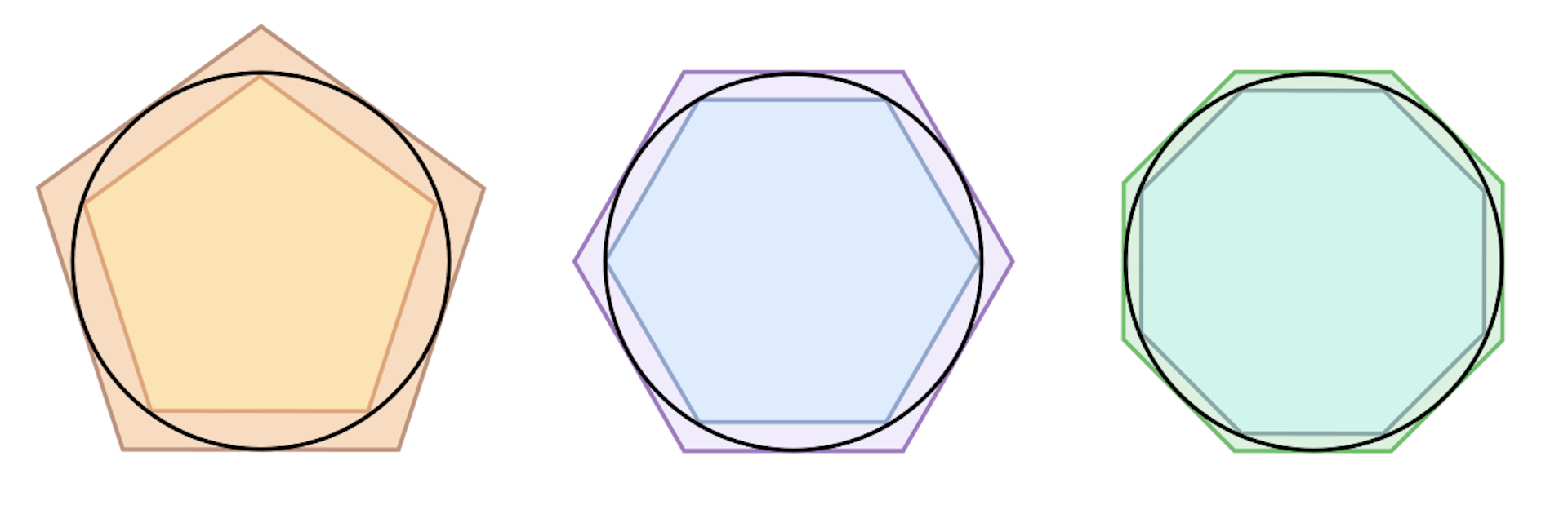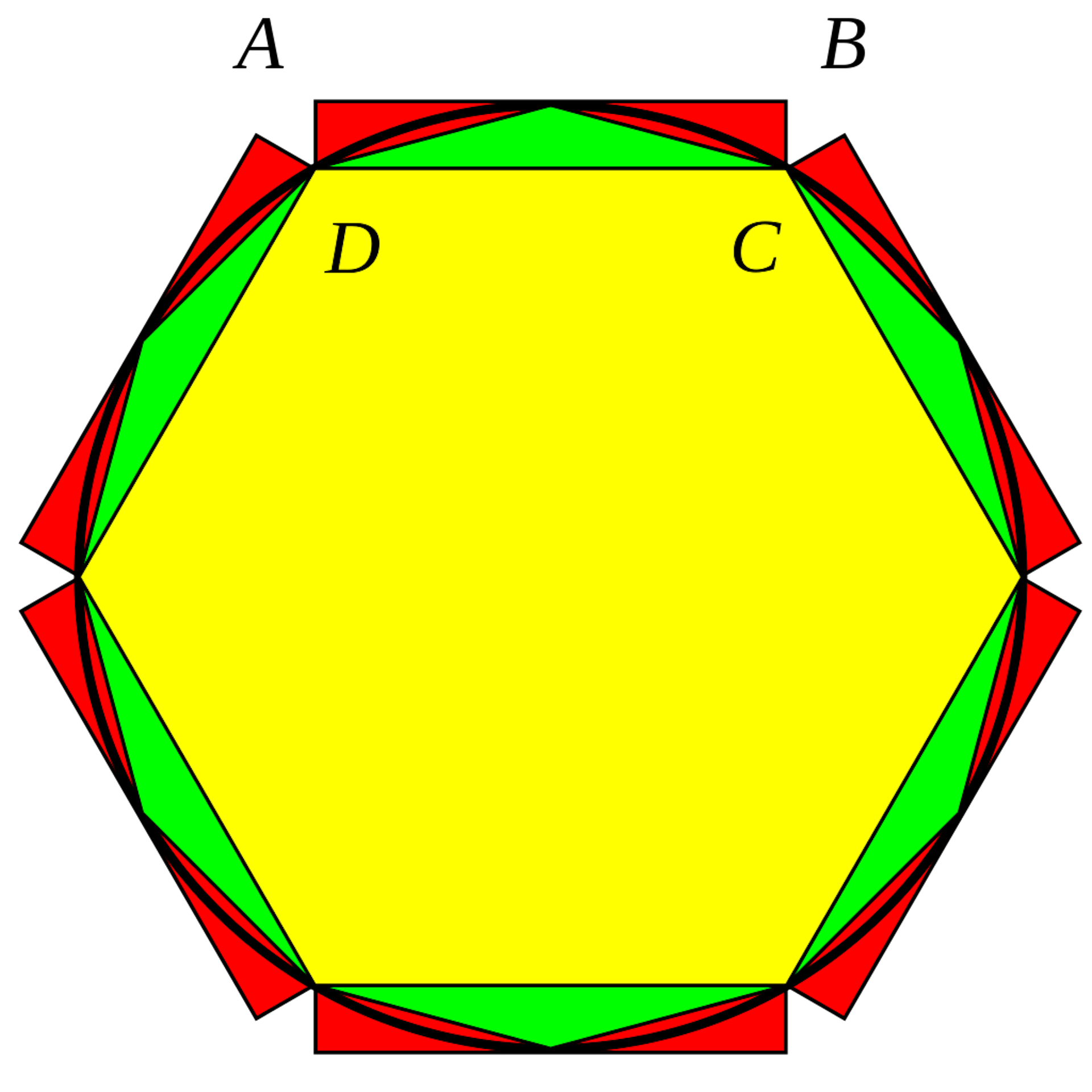# The search for the value of piThis “pi plate” shows some of the progress toward finding all the digits of pi. Piledhigheranddeeper, CC BY-SA

The number represented by pi (π) is used in calculations whenever something round (or nearly so) is involved, such as for circles, spheres, cylinders, cones and ellipses. Its value is necessary to compute many important quantities about these shapes, such as understanding the relationship between a circle’s radius and its circumference and area (circumference=2πr; area=πr2).

Pi also appears in the calculations to determine the area of an ellipse and in finding the radius, surface area and volume of a sphere.

Our world contains many round and near-round objects; finding the exact value of pi helps us build, manufacture and work with them more accurately.

Historically, people had only very coarse estimations of pi (such as 3, or 3.12, or 3.16), and while they knew these were estimates, they had no idea how far off they might be.

The search for the accurate value of pi led not only to more accuracy, but also to the development of new concepts and techniques, such as limits and iterative algorithms, which then became fundamental to new areas of mathematics.

## Finding the actual value of pi

Between 3,000 and 4,000 years ago, people used trial-and-error approximations of pi, without doing any math or considering potential errors. The earliest written approximations of pi are 3.125 in Babylon (1900-1600 B.C.) and 3.1605 in ancient Egypt (1650 B.C.). Both approximations start with 3.1 – pretty close to the actual value, but still relatively far off.Archimedes’ method of calculating pi involved polygons with more and more sides. Leszek Krupinski, CC BY-SA

The first rigorous approach to finding the true value of pi was based on geometrical approximations. Around 250 B.C., the Greek mathematician Archimedes drew polygons both around the outside and within the interior of circles. Measuring the perimeters of those gave upper and lower bounds of the range containing pi. He started with hexagons; by using polygons with more and more sides, he ultimately calculated three accurate digits of pi: 3.14. Around A.D. 150, Greek-Roman scientist Ptolemy used this method to calculate a value of 3.1416.Liu Hui’s method of calculating pi also used polygons, but in a slightly different way. Gisling and Pbroks13, CC BY-SA

Independently, around A.D. 265, Chinese mathematician Liu Hui created another simple polygon-based iterative algorithm. He proposed a very fast and efficient approximation method, which gave four accurate digits. Later, around A.D. 480, Zu Chongzhi adopted Liu Hui’s method and achieved seven digits of accuracy. This record held for another 800 years.

In 1630, Austrian astronomer Christoph Grienberger arrived at 38 digits, which is the most accurate approximation manually achieved using polygonal algorithms.

## Moving beyond polygons

The development of infinite series techniques in the 16th and 17th centuries greatly enhanced people’s ability to approximate pi more efficiently. An infinite series is the sum (or much less commonly, product) of the terms of an infinite sequence, such as ½, ¼, 1/8, 1/16, … 1/(2n). The first written description of an infinite series that could be used to compute pi was laid out in Sanskrit verse by Indian astronomer Nilakantha Somayaji around 1500 A.D., the proof of which was presented around 1530 A.D.Sir Isaac Newton. Wellcome Trust, CC BY

In 1665, English mathematician and physicist Isaac Newton used infinite series to compute pi to 15 digits using calculus he and German mathematician Gottfried Wilhelm Leibniz discovered. After that, the record kept being broken. It reached 71 digits in 1699, 100 digits in 1706, and 620 digits in 1956 – the best approximation achieved without the aid of a calculator or computer.

In tandem with these calculations, mathematicians were researching other characteristics of pi. Swiss mathematician Johann Heinrich Lambert (1728-1777) first proved that pi is an irrational number – it has an infinite number of digits that never enter a repeating pattern. In 1882, German mathematician Ferdinand von Lindemann proved that pi cannot be expressed in a rational algebraic equation (such as pi²=10 or 9pi4 - 240pi2 + 1492 = 0).

## Toward even more digits of pi

Bursts of calculations of even more digits of pi followed the adoption of iterative algorithms, which repeatedly build an updated value by using a calculation performed on the previous value. A simple example of an iterative algorithm allows you to approximate the square root of 2 as follows, using the formula (x+2/x)/2:

• (2+2/2)/2 = 1.5
• (1.5+2/1.5)/2 = 1.4167
• (1.4167+2/1.4167)/2 = 1.4142, which is a very close approximation already.

Advances toward more digits of pi came with the use of a Machin-like algorithm (a generalization of English mathematician John Machin’s formula developed in 1706) and the Gauss-Legendre algorithm (late 18th century) in electronic computers (invented mid-20th century). In 1946, ENIAC, the first electronic general-purpose computer, calculated 2,037 digits of pi in 70 hours. The most recent calculation found more than 13 trillion digits of pi in 208 days!

It has been widely accepted that for most numerical calculations involving pi, a dozen digits provides sufficient precision. According to mathematicians Jörg Arndt and Christoph Haenel, 39 digits are sufficient to perform most cosmological calculations, because that’s the accuracy necessary to calculate the circumference of the observable universe to within one atom’s diameter. Thereafter, more digits of pi are not of practical use in calculations; rather, today’s pursuit of more digits of pi is about testing supercomputers and numerical analysis algorithms.

## Calculating pi by yourself

There are also fun and simple methods for estimating the value of pi. One of the best-known is a method called “Monte Carlo.”A square with inscribed circle. Deweirdifier

The method is fairly simple. To try it at home, draw a circle and a square around it (as at left) on a piece of paper. Imagine the square’s sides are of length 2, so its area is 4; the circle’s diameter is therefore 2, and its area is pi. The ratio between their areas is pi/4, or about 0.7854.

Now pick up a pen, close your eyes and put dots on the square at random. If you do this enough times, and your efforts are truly random, eventually the percentage of times your dot landed inside the circle will approach 78.54% – or 0.7854.

Now you’ve joined the ranks of mathematicians who have calculated pi through the ages.

### Want to write?

Write an article and join a growing community of more than 89,600 academics and researchers from 2,955 institutions.